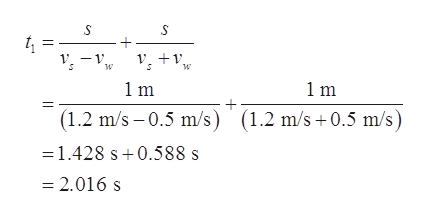# A river has a steady speed of 0.500 m/s. A student swimsupstream a distance of 1.00 km and swims back to the startingpoint. (a) If the student can swim at a speed of 1.20 m/s instill water, how long does the trip take? (b) How much time isrequired in still water for the same length swim? (c) Intuitively,why does the swim take longer when there is a current?

Question
4 views

A river has a steady speed of 0.500 m/s. A student swims
upstream a distance of 1.00 km and swims back to the starting
point. (a) If the student can swim at a speed of 1.20 m/s in
still water, how long does the trip take? (b) How much time is
required in still water for the same length swim? (c) Intuitively,
why does the swim take longer when there is a current?

check_circle

Step 1

Let vs be the students speed in still water, vw be the speed of water, s be the distance travelled, t1 be the time taken for the trip if the water has current, and t2 be the time taken for the trip if the water is still.

Write the expression for the time taken in the first case when the water is having a current.help_outlineImage Transcriptioncloset V -v +v. 1m 1m (1.2 m/s – 0.5 m/s) (1.2 m/s +0.5 m/s) =1.428 s+0.588 s = 2.016 s fullscreen
Step 2

Write the expression for the time taken in th...

### Want to see the full answer?

See Solution

#### Want to see this answer and more?

Solutions are written by subject experts who are available 24/7. Questions are typically answered within 1 hour.*

See Solution
*Response times may vary by subject and question.
Tagged in
SciencePhysics

### Kinematics